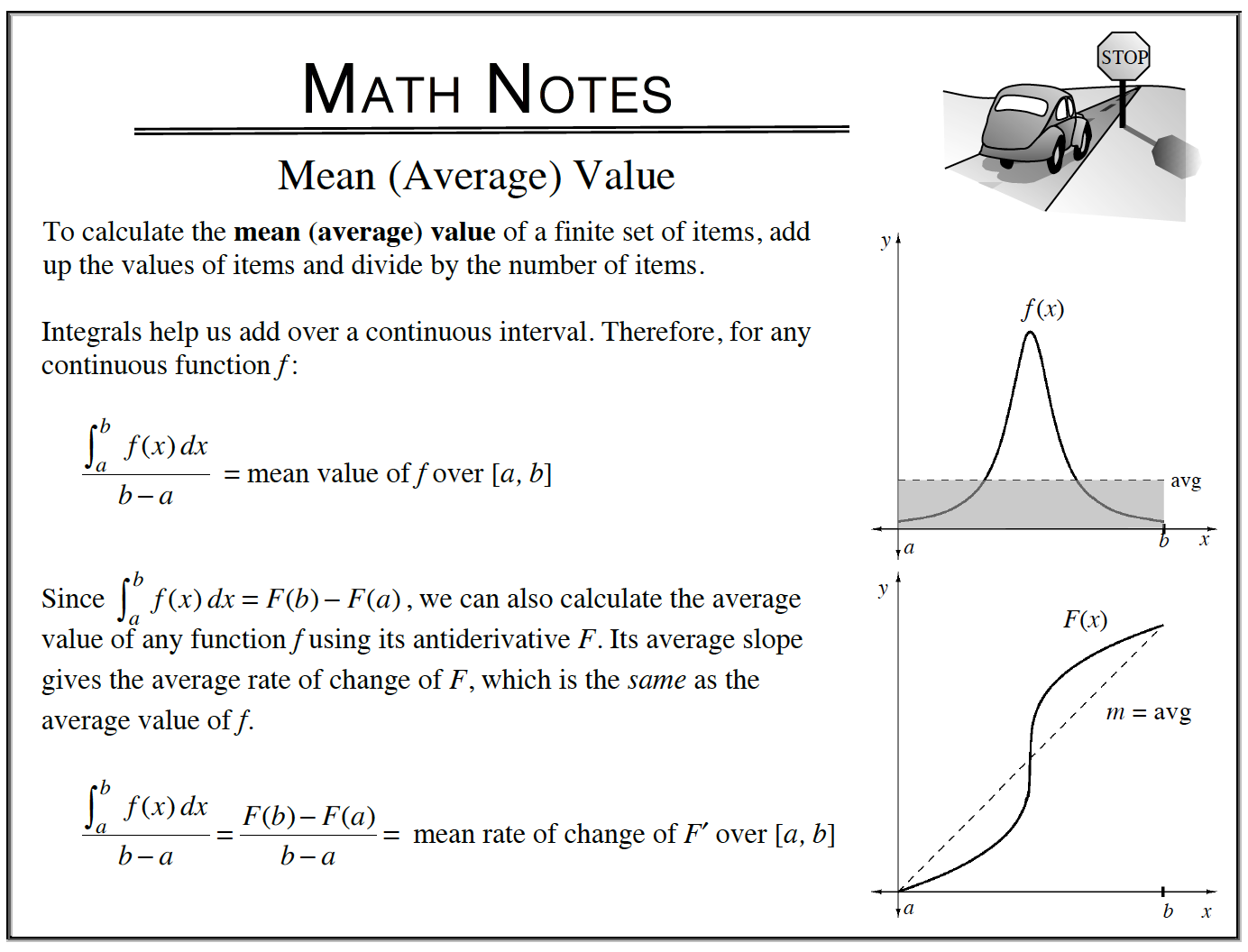### Home > APCALC > Chapter 6 > Lesson 6.4.2 > Problem6-134

6-134.

Calculate the mean value of each of function below over one complete cycle of its graph. Homework Help ✎

$\text{Average value of }f(x)=\frac{\Delta f(x)}{\Delta x}=\frac{\int_{a}^{b}f(x)dx}{b-a}$

For each of the functions below, we are given $f(x)$ and we want to find the average value of $f(x)$.1. $f(x) = \sin(x)$

$y = \sin(x)$ has a period of $2π$.
So choose a and b-values such that $Δx = 2π$.

2. $g(x) =| \operatorname { sin } ( x ) |$

Compare the periods of $y = |\sin(x)|$ and $y = \sin(x)$.

3. $k(x) = \sin^2(x)$

Did you realize that since

$\cos\Big(t-\frac{\pi}{2}\Big)=\sin(t), \sin^2(t)=\cos^2\Big(t-\frac{\pi}{2}\Big)=\cos^2(t)?$

$\int_{0}^{2\pi}\sin^2(x)dx=\frac{1}{2}\int_{0}^{2\pi}\sin^2(x)+\cos^2(x)dx$

$\frac{\int_{0}^{2\pi}g(x)dx}{2\pi - 0}=\frac{1}{2}$